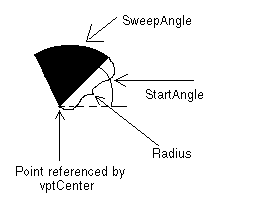﻿ VECTORCHORD | Vector Structures | Vector C++ Class Library Help
In This Topic ▼

# VECTORPIE/VECTORCHORD

````typedef struct tagVECTORPIE `
`{ `
`   VECTOROBJECT Object; `
`   VECTORPOINT Point; `
`   VECTORPEN Pen; `
`   VECTORBRUSH Brush; `
`   L_DOUBLE Radius; `
`   L_DOUBLE StartAngle; `
`   L_DOUBLE SweepAngle; `
`} VECTORPIE, * pVECTORPIE, VECTORCHORD, * pVECTORCHORD; ````

The VECTORPIE structure holds information about a vector arc object.

The VECTORCHORD structure holds information about a vector chord object.

## Members

Abstract object.

### VECTORPOINT Point

A VECTORPOINT structure that contains the vertex of a vector pie object, about which the pie section or chord sweeps.

### VECTORPEN Pen

VECTORPEN structure that contains information about the pen to use when drawing the vector pie or vector chord object.

### VECTORBRUSH Brush

VECTORBRUSH structure that contains information about the brush to use when drawing the vector pie or vector chord object.

Length of the radius of the vector pie or vector chord object. This value is in logical units.

### L_DOUBLE StartAngle

The angle at which to begin the vector pie or vector chord. Values should be between 0 and 360 degrees. Values less than 0 and greater than 360 will be modified internally.

### L_DOUBLE SweepAngle

The angle through which to sweep the vector pie or vector chord. Values should be between 360 and +360 degrees. Positive angles will be drawn in a counter-clockwise direction from StartAngle. Negative angles will be drawn in a clockwise direction.

nSize of the Object member must be the sizeof this structure.

nType of the Object member must be VECTOR_PIE or VECTOR_CHORD.

pVECTORPIE is a pointer to a VECTORPIE structure.

pVECTORCHORD is a pointer to a VECTORCHORD structure.

The diagram below gives a graphical representation of the Point, Radius, StartAngle and SweepAngle parameters.Help Version 21.0.2021.4.7